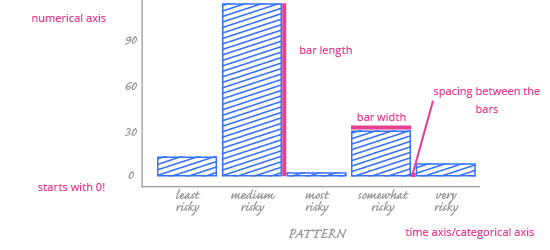Deals Of The Week - hours only!Up to 80% off on all courses and bundles.-Close
What we will learn
Visualize your data - bar chart
Check yourself
18. Showing Change Over Time with a Bar Chart - Summary

## Instruction

Congratulations! In this chapter, we talked about visualizing change over time.

You’ve completed all the exercises in this part, but let’s have a summary before we move on.

Time has a dual nature: it can represent one exact moment (like one minute) or a longer period (like a decade). In data visualization, we can interpret time variables in one of two ways:

• If the variable represents a period of time (e.g. a decade), we treat it as a categorical ordinal variable.
• If the variable represents a point in time (e.g. a minute), we treat it as a numerical variable.

To visualize change over time, we need time-series data. A time series is a dataset that contains two columns:

• A numerical variable, where each value represents something at a specific moment in time, and
• A time variable, which tells us when the numerical value was recorded.

We can use bar charts to visualize change over time.When you’re using a time variable categorically – that is, when the variable represents periods of time – bar charts are the natural choice to visualize time-series data. Why?

• Readers can quickly grasp the magnitude of numerical values for each period.
• It’s also easy to understand the difference between values.

Follow these best practices when using a bar chart to show change over time:

• Always start the y-axis at 0, even for time-series data.
• Adding color to your bars will make the data stand out. Remember, though, that your goal is to highlight the story, not decorate the chart!
• Don’t use a bar chart to show trends over time – reading trends from a bar chart is hard.

Next up, we’ll discuss another chart type used to visualize change over time: the line chart.